# A Magical Theorem, Part II: Areas by way of Antiderivatives

So how are areas related to antiderivatives?  In other words (and symbols):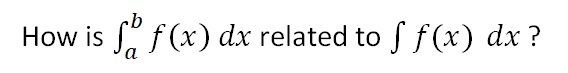This is the fundamental question.  When you know the answer, you will know the “Fundamental Theorem of Integral Calculus”.

In your first physics class, while studying kinematics, you learned that the area under a velocity vs time graph tells us the change in the object’s position.  To help jog your memory, play the video below:

The upper graph shows the velocity as a function of time for an object that happened to have a constant velocity of 10 m/s.

The lower graph shows the position vs time for the same object.  The velocity is constant so the position graph is linear with a constant slope.

On the velocity graph, you can see the area being swept out from t = 3 s to t = 7 s.  And on the position graph, you can see how far the object has moved during the same time interval.  Try stopping the animation at different times. Examine the graphs until you have convinced yourself of the following claim:

For a given time interval, the area under the Velocity vs. time graph will be equal to the change in position during that time interval.

YOU MAY BE SKEPTICAL…

After all, that was just with constant velocity.  But what if the velocity is changing?  Like so…

The velocity is increasing linearly and the position is increasing quadratically. [Remember, velocity is the derivative of position with respect to time. And the derivative of a 2nd-degree polynomial is a linear function.]

But even so, once again we see:

For a given time interval, the area under the Velocity vs. time graph will be equal to the change in position during that time interval.

STILL SKEPTICAL? STAY TUNED…

The velocity does not have to be changing linearly.  In fact, it does not even have to be velocity and position that we are talking about.  It can be any two quantities where one is the derivative of the other. Here’s one where the first graph is the rate at which water flows into a container and the second graph is the volume of water in that container:

Stop the animation at various points to check our claim:

If f(x) is the derivative of F(x), then the area under the graph of f(x) is equal to the change in F(x) over that same interval.

And that answers the question we started with. What does area have to do with antiderivatives?  The area under the graph is equal to the change in the antiderivative.  That is called the Fundamental Theorem.  We write: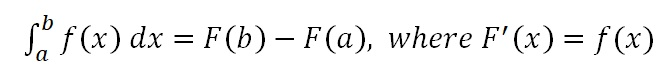WHY IS THIS USEFUL?

Well, suppose you want to find the area under some graph. This theorem gives you a procedure you can follow:

Step 1: Find an antiderivative.

Step 2. See how much the antiderivative changed by over the interval.

For example, suppose we want to know the area under the graph of f(x)=x2 between x=1 and x = 2, as illustrated below.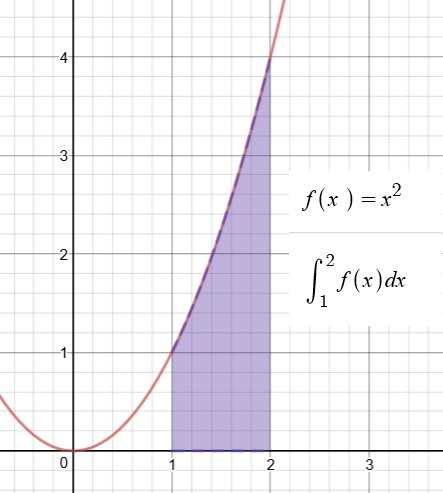Let’s see.  Can we find an antiderivative?  Oh, yes, this one we did already.

F(x)=(1/3)x3 is an antiderivative of  f(x)=x2.

So now we just have to see how much F(x) has changed over the interval from x = 1 to x = 3.

F(3) = (1/3)(2)3 = 8/3

F(1) = (1/3)(1)3 = 1/3

F(3) – F(1) = 7/3

But what if I can’t find the antiderivative?

Well, that could be a problem.  That’s why you will spend so much time developing this skill in AP Calculus.  But I will reassure you again: in AP Physics, the antiderivatives you need will be manageable.  And there’s always Wolfram Alpha

I still don’t see why this is so important?  Are there lots of areas to be found in AP Physics?

Hmm.. No and yes.  See next post…

# A Magical Theorem, Part I: Antiderivatives and Areas

If you have been working your way through these Summer Reading posts, then at this point, you know how to find “derivatives” for a nice collection of reasonable functions.  Nothing too crazy or exotic — just the kinds of functions that you will meet early in AP Physics C.  And you also know why we find derivatives:  derivatives are slope-finding formulas.

For example:

Velocity is the rate of change of position with respect to time.

Acceleration is the rate of change of velocity with respect to time.

Force is the rate of change of momentum with respect to time.

Current is the rate of change of electric charge with respect to time.

For example, suppose you are given that an object moves so that it’s velocity as a function of time is:

v(t) = t2

You can easily find the acceleration as function of time by taking the derivative:

a(t) = v’(t) = 2t

So there are good, useful reasons for learning how to find derivatives. (In fact, let me remind you that to “optimize” a function, you usually begin by finding the derivative and then setting it equal to zero.)

CAN WE PLAY THE GAME IN REVERSE?

If I give you a function, can you find an “antiderivative”?

For example, let’s say:  f(x)=x2

Can we find another function that has f(x)=x2 as its derivative? If we know the basics, finding this particular antiderivative is not that hard:

F(x)=(1/3)x3

But you don’t have to take my word — go ahead an take the derivative!  You will see for yourself that the derivative of F(x)=(1/3)x3 is indeed f(x)=x2.

So  F(x)=(1/3)x3 is an antiderivative of  f(x)=x2.

But it is not the only one!  Try taking the derivative of any of these:

F(x)=(1/3)x3 + 5

F(x)=(1/3)x3 – 99

F(x)=(1/3)x3 + C where C is a constant

So if you can find one antiderivative, you can find a whole family of them.  Still, most times, you only need to find one.

[Caution: your math teacher will likely take off a point or two if you leave out the “…+ c” when finding an antiderivative.]

IS IT ALWAYS THIS EASY?

Well, no.  Some of them are easy.  But others…not so much.  You will spend a substantial amount of time in math class building a collection methods for finding antiderivatives for an increasing variety of functions.  And some of these methods are quite subtle.  For now, I want to make two points:

In our AP Physics class, when the antiderivatives are hard to find, you can have a little help: your TI89s or N-Spires know how to do this.  So does Wolfram Alpha.

Nobody would be willing to invest so much effort in finding antiderivatives unless there was something really useful about them!

A NEW NOTATION

If we are going to be using antiderivatives, we should have symbols for writing them.  To indicate that F(x) is the antiderivative of f(x), we write:This is just another way of saying that F'(x) =  f(x).  And, in addition to calling F(x)  an antiderivative, we also sometimes call it the “indefinite integral of f(x)”.

BUT WAIT…

On the other hand, there is another symbol that looks almost the same but means something completely different: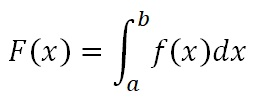This symbol is called the “definite integral” (as opposed to “indefinite”).  It is not a function at all! Just adding values at the top and bottom of that strange elongated s-shape gives the symbol a whole new meaning:

The definite integral is the area of the region bounded by the function, the x-axis and the two “limits of integration”: the vertical lines defined by x=a and x=b.

For example, here is a definite integral: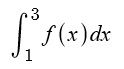And here is the area that definite integral is referring to: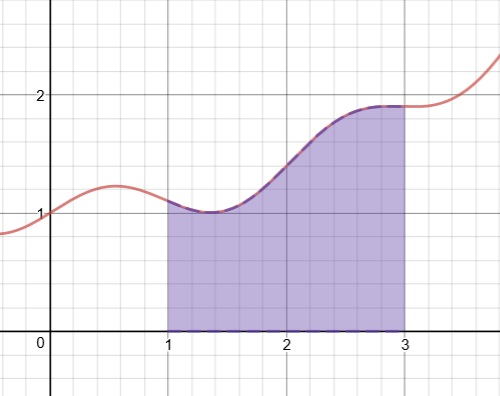So now we have two very different ideas that have been given very similar symbols and names. It would be easy to get these two things confused…

There is the INDEFINITE INTEGRAL:This is a FUNCTION which has f(x) as its derivative.

And then there is the DEFINITE INTEGRAL:This is an AREA — a numeric value.

And now the big question:

Why do mathematicians use these similar names and symbols for these two very different ideas?  Are they secretly related in some FUNDAMENTAL* way?

That is the topic of the next post.